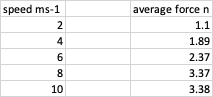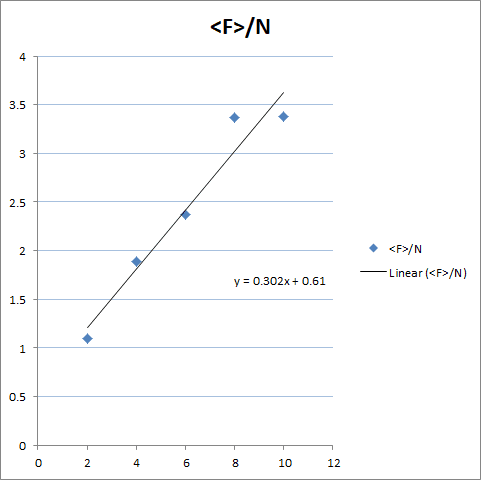# Finding the coefficient of viscosity

joshm12
Homework Statement:
How do I work out the coefficient of viscosity when the slope of a graph =0.34
n= coefficient of viscosity
v=speed of rotation
f= force required to maintain speed
Relevant Equations:
F=1.67nv
"Calculate the gradient (or slope) of the line and use this and the equation above to calculate the value for the viscosity, η. You MUST use the gradient to calculate the viscosity." here are the results:I'm not sure if it's just me who finds this confusing

Homework Helper
Hello @joshm12,!

What did you find for the slope of the line ? And how did you do that ? By forcing an intercept 0 ?
Did you make a plot to see if that is justified ?

Are these your own measurements or were they given as part of the problem statement ?

joshm12
@BvU I used the change in y/change in x to find the slope of the line and got 0.34. and the measurements were given as part of the problem

Last edited:
Homework Helper
I used the change in y/change in x
Yes, I know that. How did you do it ?

Mentor
This is for flow between a rotating cylinder and a stationary cylinder. Is the gap between the cylinders small?

Homework Helper
What did you find for the slope of the line ? And how did you do that ? By forcing an intercept 0 ?
Did you make a plot to see if that is justified ?